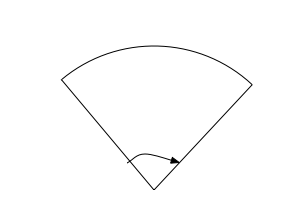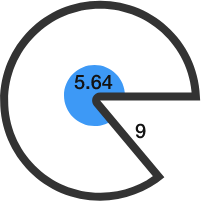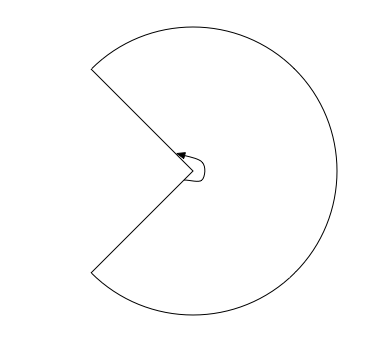Geometry

# Circles - Arc LengthIn the above sector, the inscribed angle is $0.68$ radians and the radius is $4$. What is the arc length of the sector?In the above sector, the angle is $0.84$ radians and the radius is $5$. What is the perimeter of the sector?

A circular sector of radius 8 has an arc length of 32. What is the angle (in radians) of the sector?In the above sector, the marked angle is $5.64$ radians and the radius is $9$. What is the perimeter of the sector?In the above sector, the inscribed angle is $5.64$ radians and the radius is $9$. What is the arc length of the sector?

×